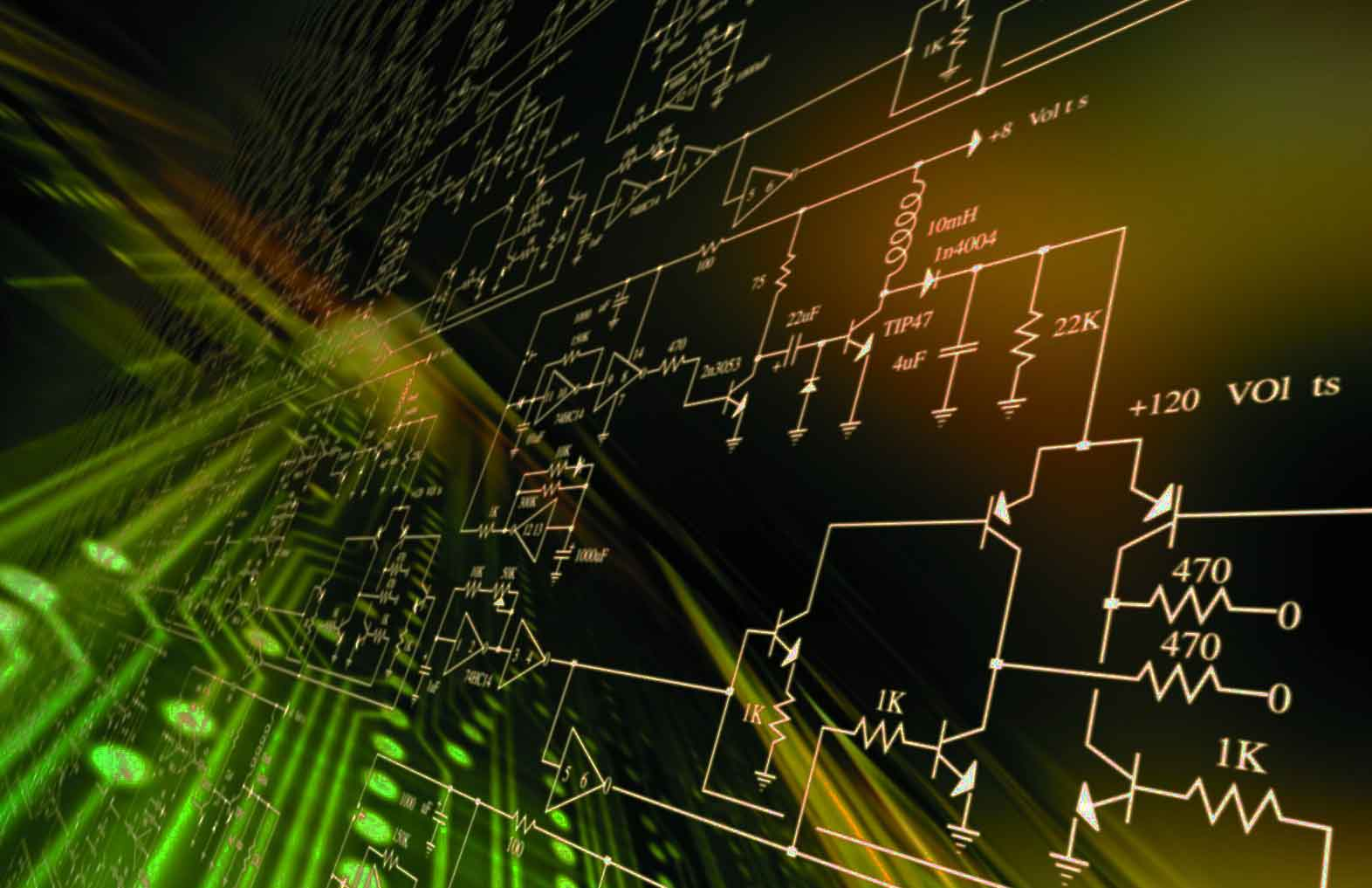# MATLAB Simulations using Simulink for Power Electronics, Electric Motors, Generators and Solar

Learn how to use MATLAB/Simulink for power electronics circuits, electric circuits, electrical machines, solar and synchronous generators. 47 lessons in 13h 52m total course length.

Current Status
Not Enrolled
Price
\$23.45
Get Started

## Course Description

Learn the leading software MATLAB in numerical computing through step-by-step exercises. In this course, we will learn to simulate AC-DC, DC-AC, AC-AC, and DC-DC converter circuits, electric circuits, electrical machines, solar and synchronous generators in the MATLAB using Simulink tool circuits.

In this course, you will learn the simulation using MATLAB of:

• Single-phase half-wave controlled rectifier
• Model the DC machine in no-load case
• Single-phase bridge controlled rectifier
• Model the DC machine in presence of load torque
• Single-phase AC chopper with R and RL load
• Simulating the DC machine using power library
• A DC-DC converter as buck regulator
• Three-phase Inverter
• Boost regulator
• Single-phase half-wave controlled rectifier
• Buck-Boost regulator
• Induction motor
• Single-phase half-bridge inverter
• PV cell in solar energy
• Single-phase and three-phase bridge inverter
• PID controller
• Single-phase half-wave controlled rectifier
• Analyzing data from the PSCAD program

## Course Summary

1. Applications on Matrices in MATLAB
2. Power Electronics Simulations
3. Solar Energy Simulation
4. DC Motor Simulation
5. Induction Motor Simulation
6. Synchronous Generator Simulation
7. Power System Simulations
8. PID Controller in MATLAB

## Who Is This Course For

• Engineering students
• Practicing engineers
• Anybody with an interest in learning about power electronics and MATLAB/Simulink

## Requirements

MATLAB/Simulink software, free trial available online

1. Matlab Simulations (PDF)
2. Fault Location Matlab + PSCAD (ZIP)

## Course Content

##### Ahmed Mahdy

I am an electrical power engineer, and I am also an electrical instructor at more than 30 platforms, teaching electrical engineering to more than 60,000 students from more than 170 countries. I also work in the electrical research field on topics related to power system stability, renewable energy systems, electric vehicles, and control systems.

11 Courses+22 enrolled
Not Enrolled

#### Course Includes

• 8 Lessons
• 47 Topics
• Course Certificate